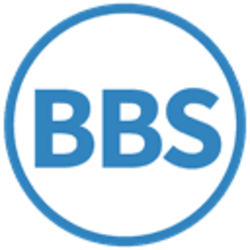# Almost

Use Wikipedia with dynamical search help in all languages ...

Wikipedia - How to create a pageIn set theory, when dealing with sets of infinite size, the term almost or nearly is used to refer to all but a negligible amount of elements in the set. The notion of "negligible" depends on the context, and may mean "of measure zero" (in a measure space), "finite" (when infinite sets are involved), or "countable" (when uncountably infinite sets are involved).

For example:

• The set $S=\{n\in \mathbb {N} \,|\,n\geq k\}$is almost $\mathbb {N}$for any $k$in $\mathbb {N}$, because only finitely many natural numbers are less than $k$.
• The set of prime numbers is not almost $\mathbb {N}$, because there are infinitely many natural numbers that are not prime numbers.
• The set of transcendental numbers are almost $\mathbb {R}$, because the algebraic real numbers form a countable subset of the set of real numbers (which is uncountable).
• The Cantor set is uncountably infinite, but has Lebesgue measure zero. So almost all real numbers in (0, 1) are members of the complement of the Cantor set.This page is funded by cryptomining